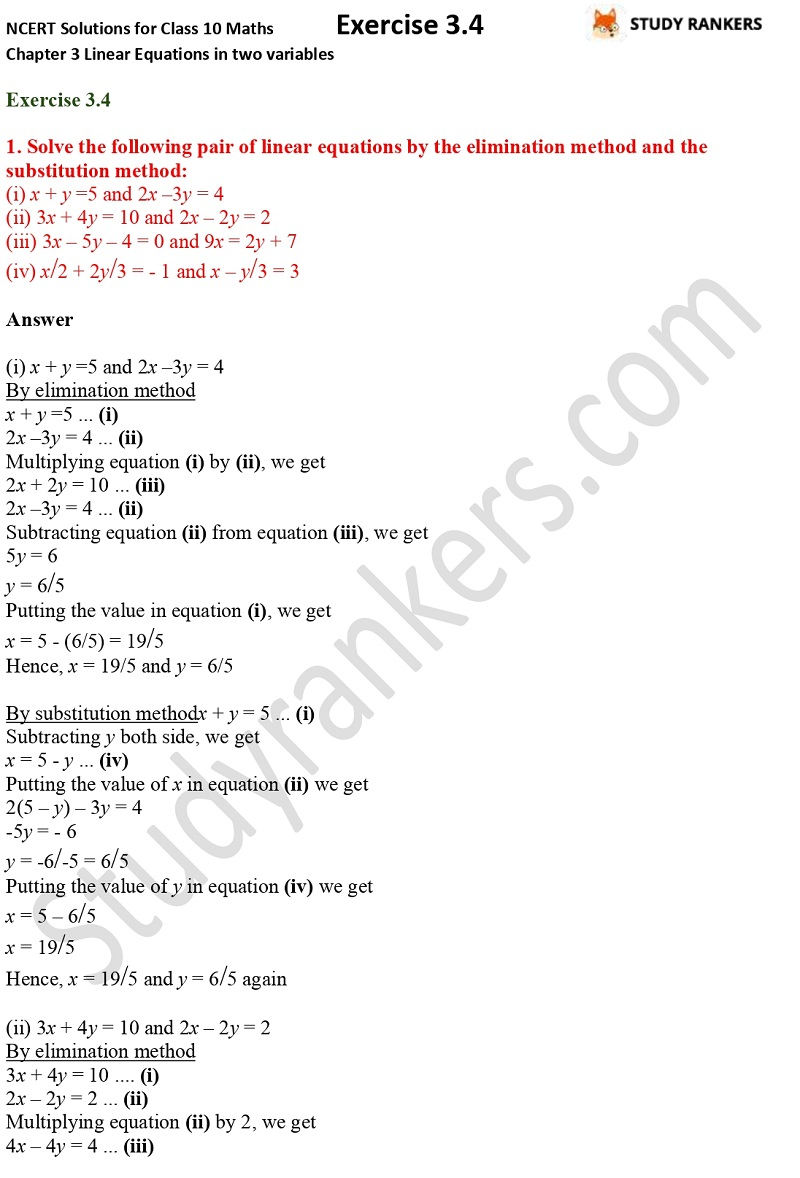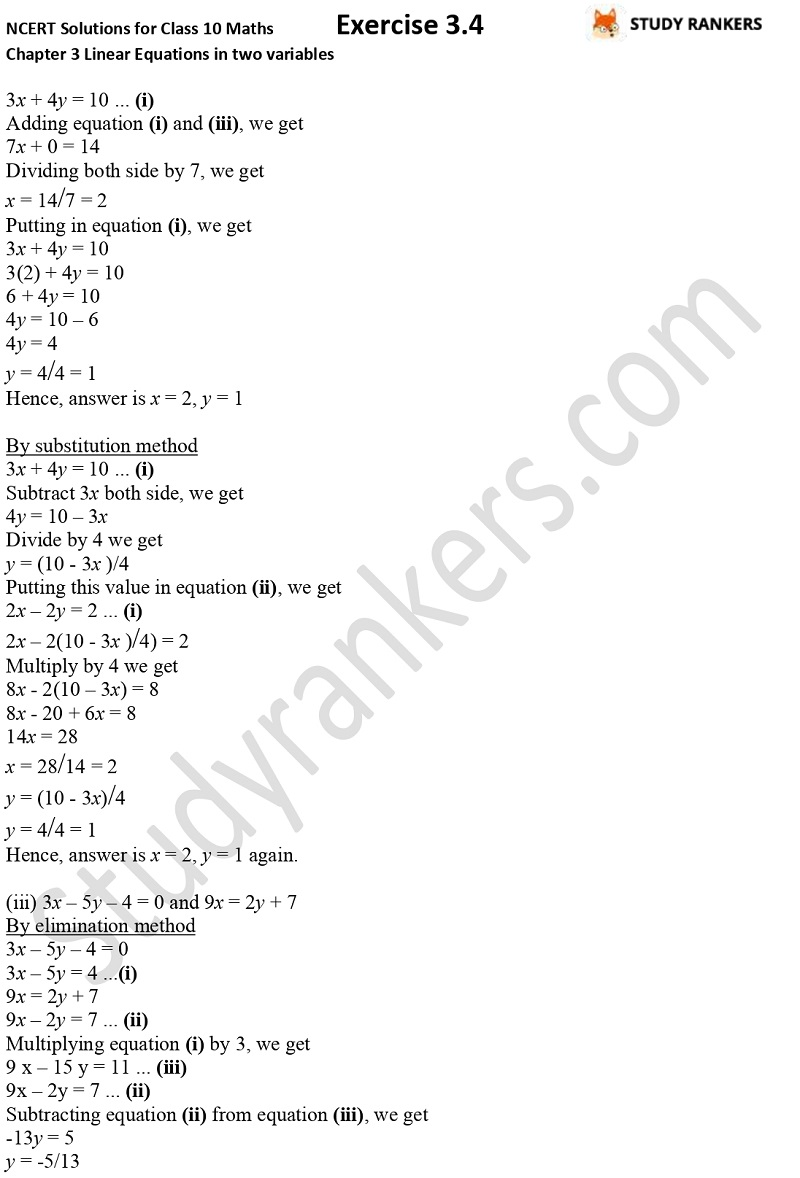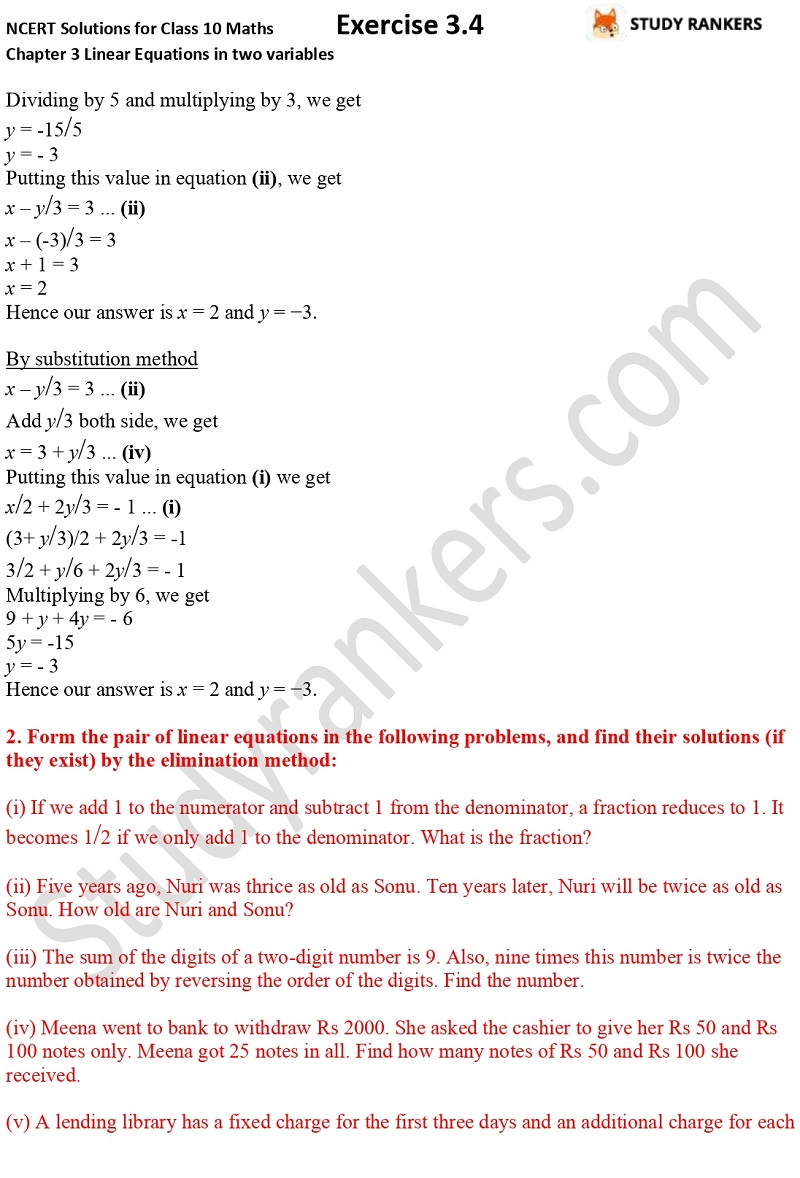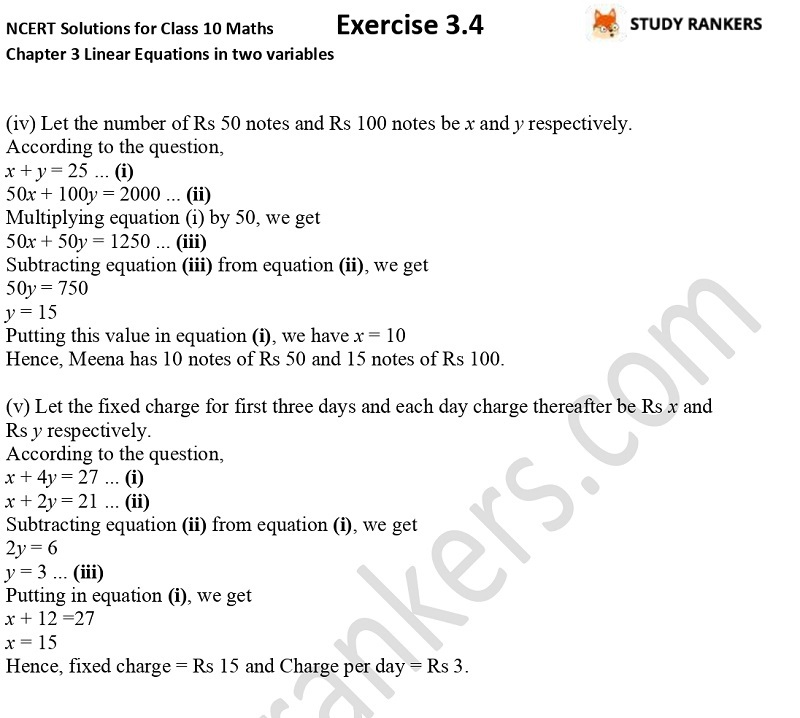## NCERT Solutions for Class 10 Maths Chapter 3 Pair of Linear Equations in Two Variables Exercise 3.4

If you're in search of NCERT Solutions for Class 10 Maths Chapter 3 Pair of Linear Equations in Two Variables Exercise 3.4 then you're at right place. Here we have provided CBSE NCERT Solutions based on latest NCERT textbook. These will help you building your basics and performing better in maths exams. There are total two questions in the exercise. In the first you have to solve pair of linear equations by the elimination method and the substitution method and in the other one you have to form the pair of linear equations and find their solutions by the elimination method.